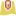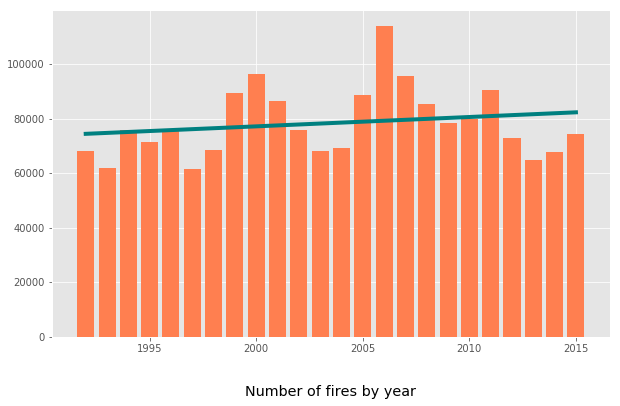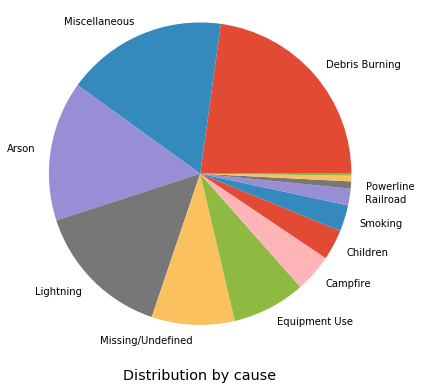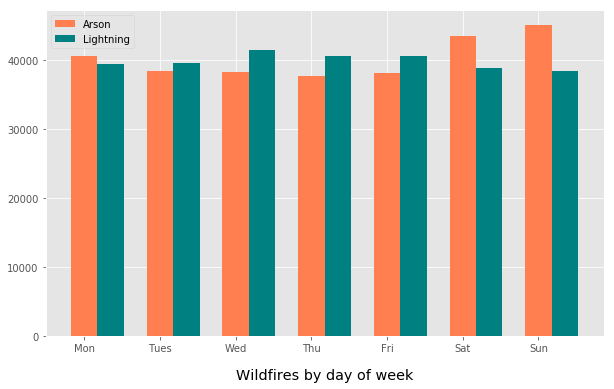ArcGIS Developers
Dashboard

Historical Wildfire Analysis¶

Spatial wildfire occurrence data, referred to as the Fire Program Analysis fire-occurrence database (FPA FOD), for the United States, 1992-2015 was obtained from https://www.fs.usda.gov/rds/archive/Product/RDS-2013-0009.4/. The dataset contains data on:

• 1.88 Million US Wildfires
• 24 years of geo-referenced wildfire records

Given this data, can we answer the following questions?¶

• Are wildfires increasing over time?
• Where are the fire hot spots?
• Can Machine Learning categorize the cause of the fire given the size, location and date?

That would give investigators a data driven way to prioritize cases for further investigation.

In :
from arcgis import *
import pandas as pd
import numpy as np
In :
gis = GIS(profile='deldev.maps')
In :
from arcgis.features import GeoAccessor, GeoSeriesAccessor

wildfires_df = pd.DataFrame.spatial.from_featureclass(r'C:\demo\FPA_FOD_20170508.gdb\Fires')
In :
wildfires_df.shape
Out:
(1880465, 39)
In :
df = wildfires_df[['FIRE_YEAR', 'DISCOVERY_DATE', 'DISCOVERY_DOY',
'STAT_CAUSE_DESCR', 'FIRE_SIZE', 'STATE', 'FIPS_CODE', 'FIPS_NAME', 'SHAPE']]
In :
Out:
FIRE_YEAR DISCOVERY_DATE DISCOVERY_DOY STAT_CAUSE_DESCR FIRE_SIZE STATE FIPS_CODE FIPS_NAME SHAPE
0 2005 2005-02-02 33 Miscellaneous 0.10 CA 063 Plumas {'x': -121.00583333000003, 'y': 40.03694443999...
1 2004 2004-05-12 133 Lightning 0.25 CA 061 Placer {'x': -120.40444444000002, 'y': 38.93305556000...
2 2004 2004-05-31 152 Debris Burning 0.10 CA 017 El Dorado {'x': -120.73555555999997, 'y': 38.98416666999...
3 2004 2004-06-28 180 Lightning 0.10 CA 003 Alpine {'x': -119.91333333, 'y': 38.55916666999997, '...
4 2004 2004-06-28 180 Lightning 0.10 CA 003 Alpine {'x': -119.93305555999996, 'y': 38.55916666999...

Exploratory Data Analysis¶

In :
df['x'] = df.SHAPE.apply(lambda g: g['x'])
df['y'] = df.SHAPE.apply(lambda g: g['y'])
smdf = wildfires_df[['FIRE_YEAR', 'DISCOVERY_DATE', 'DISCOVERY_DOY',
'STAT_CAUSE_DESCR', 'FIRE_SIZE', 'STATE', 'FIPS_CODE', 'FIPS_NAME', 'x', 'y']]
smdf.to_pickle('fires.pkl')
In :
pd.options.mode.chained_assignment = None

df['MONTH'] = pd.DatetimeIndex(df['DISCOVERY_DATE']).month
df['DAY_OF_WEEK'] = df['DISCOVERY_DATE'].dt.dayofweek
df_arson = df[df['STAT_CAUSE_DESCR']=='Arson']
dfa = df_arson['DAY_OF_WEEK'].value_counts()
df_lightning = df[df['STAT_CAUSE_DESCR']=='Lightning']
dfl = df_lightning['DAY_OF_WEEK'].value_counts()
In [ ]:
%matplotlib inline
import matplotlib.pyplot as plt

plt.style.use('ggplot')
sorted_df = df.sort_values('FIRE_YEAR')
fire_freq = sorted_df.groupby('FIRE_YEAR').count()
x = np.asarray(fire_freq.axes)
y = np.asarray(df.groupby('FIRE_YEAR').size())
In :
lyr = gis.content.search('Demographic Profile')
lyr
Out:
Demographic ProfileFeature Layer Collection by deldev

Have wildfires become more frequent over time?¶

In :
fig, ax = plt.subplots(figsize=(10,6))
ax.bar(x, y, color='coral')
plt.title('Number of fires by year', y=-0.2)
fit1 = np.polyfit(x, y, deg=1)
ax.plot(x, x*fit1 + fit1, color='teal', linewidth=4);What are the causes of wildfire?¶

In :
distribution = df['STAT_CAUSE_DESCR'].value_counts()
In :
plt.figure(figsize=(6,6))
plt.title('Distribution by cause', y=-0.15)
plt.pie(distribution, labels=list(distribution.index[:-2]) + ['', '']);
plt.axis('equal');Does the day of week matter?¶

In :
ind = np.arange(7)
width = 0.35

fig, ax   = plt.subplots(figsize=(10,6))
arson     = ax.bar(ind, dfa.sort_index(), width, color='coral')
lightning = ax.bar(ind + width, dfl.sort_index(), width, color='teal')

ax.set_title('Wildfires by day of week', y=-0.15)
ax.set_xticklabels(('', 'Mon', 'Tues', 'Wed', 'Thu', 'Fri', 'Sat', 'Sun'))
ax.legend((arson, lightning), ('Arson', 'Lightning'), loc=2);Mapping and Spatial Analysis¶

In [ ]:
wildfires = gis.content.import_data(wildfires_df)
In [ ]:
from arcgis.features.analysis import find_hot_spots
hotspots = find_hot_spots(wildfires, 20, 'Miles', output_name='Hot Spots US_Historical_Wildfires_20')
In :
In :
usmap = gis.map('United States')
usmap

Can Machine Learning categorize the cause of the fire?¶

Since arson is a significant preventable cause for many wildfires, it would be instructive to see if Machine Learning techniques can be used to classify if a fire was caused due to arson given some basic data that is available when the fire is first discovered or when investigations begin. This can be framed as a binary classification problem.

Such a technique could give investigators a data driven way to prioritize cases for further investigation.

In :
from sklearn.model_selection import train_test_split
from sklearn import tree, preprocessing
import sklearn.ensemble as ske
import pandas as pd
import matplotlib.pyplot as plt

%matplotlib inline
%config IPCompleter.greedy=True
In :

df['MONTH'] = pd.DatetimeIndex(df['DISCOVERY_DATE']).month
df['DAY_OF_WEEK'] = df['DISCOVERY_DATE'].dt.dayofweek

ca_fires = df[df.STATE=='CA']
ca_fires['ARSON'] = ca_fires['STAT_CAUSE_DESCR'].apply(lambda x: 1 if x == 'Arson' else 0)

pdf = ca_fires[ ['FIRE_YEAR', 'DISCOVERY_DOY', 'FIPS_CODE', 'FIRE_SIZE', 'x', 'y', 'MONTH', 'DAY_OF_WEEK', 'ARSON'] ]
pdf = pdf.dropna()

Feature Engineering: Use geoenrichment to add features¶

In :
analysis_variables = [
'TOTPOP_CY',  # 2016 Population: Total Population (Esri)
'HHPOP_CY',   # 2016 Household Population (Esri)
'FAMPOP_CY',  # 2016 Family Population (Esri)
'DIVINDX_CY', # 2016 Diversity Index (Esri)
'TOTHH_CY',   # 2016 Total Households (Esri)
'AVGHHSZ_CY', # 2016 Average Household Size (Esri)

'MALES_CY',   # 2016 Gender: Male Population (Esri)
'FEMALES_CY', # 2016 Gender: Female Population (Esri)

'MEDAGE_CY',  # 2016 Age: Median Age (Esri)

'AVGFMSZ_CY', # 2016 Income: Average Family Size (Esri)
'MEDHINC_CY', # 2016 Income: Median Household Income (Esri)
'AVGHINC_CY', # 2016 Income: Average Household Income (Esri)

'EDUCBASECY', # 2016 Educational Attainment Base (Esri)
'NOHS_CY',    # 2016 Education: Less than 9th Grade (Esri)
'SOMEHS_CY',  # 2016 Education: 9-12th Grade/No Diploma (Esri)
'HSGRAD_CY',  # 2016 Education: High School Diploma (Esri)
'GED_CY',     # 2016 Education: GED/Alternative Credential (Esri)
'SMCOLL_CY',  # 2016 Education: Some College/No Degree (Esri)
'ASSCDEG_CY', # 2016 Education: Associate's Degree (Esri)
'BACHDEG_CY', # 2016 Education: Bachelor's Degree (Esri)
]
In :
from arcgis.geoenrichment import *

usa = Country.get('US')
counties = usa.subgeographies.states['California'].counties

enrich_df = enrich(list(counties.values()), analysis_variables=analysis_variables)

pdf['StdGeographyID'] = '06' + pdf['FIPS_CODE']
merged = pd.merge(pdf, enrich_df, on='StdGeographyID')
cols = ['FIRE_YEAR', 'DISCOVERY_DOY', 'FIPS_CODE', 'FIRE_SIZE', 'x', 'y', 'MONTH', 'DAY_OF_WEEK', 'ARSON'] + analysis_variables
mdf = merged[cols]

Out:
FIRE_YEAR DISCOVERY_DOY FIPS_CODE FIRE_SIZE x y MONTH DAY_OF_WEEK ARSON TOTPOP_CY ... MEDHINC_CY AVGHINC_CY EDUCBASECY NOHS_CY SOMEHS_CY HSGRAD_CY GED_CY SMCOLL_CY ASSCDEG_CY BACHDEG_CY
0 2005 33 063 0.1 -121.005833 40.036944 2 2 0 19292 ... 45275 66596 14802 447 909 3153 415 4803 1672 2048
1 2005 153 063 2.3 -120.451111 39.817222 6 3 0 19292 ... 45275 66596 14802 447 909 3153 415 4803 1672 2048
2 2005 234 063 0.5 -121.147500 39.872222 8 0 0 19292 ... 45275 66596 14802 447 909 3153 415 4803 1672 2048
3 2005 241 063 0.1 -120.903889 39.962222 8 0 0 19292 ... 45275 66596 14802 447 909 3153 415 4803 1672 2048
4 2005 242 063 1.0 -120.706667 40.056944 8 1 0 19292 ... 45275 66596 14802 447 909 3153 415 4803 1672 2048

5 rows × 29 columns

In [ ]:
m = gis.map('California')
m
In :
lyr = gis.content.import_data(enrich_df, title='Demographic Profile')
In :

Fit Random Forest classifier and check accuracy¶

In :
X = mdf.drop(['ARSON'], axis=1).values
y = mdf['ARSON'].values
X_train, X_test, y_train, y_test = train_test_split(X,y,test_size=0.3, random_state=42)

clf_rf = ske.RandomForestClassifier(n_estimators=200)
clf_rf = clf_rf.fit(X_train, y_train)

print(clf_rf.score(X_test, y_test))
0.9212070907689571

Summary: Given some basic data, available when a fire is first discovered, it is possible to predict with over 90% accuracy if the fire was the result of arson.

Citations

Short, Karen C. 2017. Spatial wildfire occurrence data for the United States, 1992-2015 [FPA_FOD_20170508]. 4th Edition. Fort Collins, CO: Forest Service Research Data Archive. https://doi.org/10.2737/RDS-2013-0009.4

Feedback on this topic?